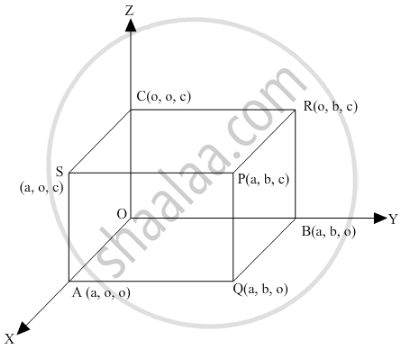Share

If a Line Makes Angles α, β, γ, δ with Four Diagonals of a Cube, Then Cos2 α + Cos2 β + Cos2 γ + Cos2 δ is Equal to 1 /3,2/ 3, 4/3,8/3. - CBSE (Arts) Class 12 - Mathematics

ConceptDirection Cosines and Direction Ratios of a Line

Question

If a line makes angles α, β, γ, δ with four diagonals of a cube, then cos2 α + cos2 β + cos2γ + cos2 δ is equal to

• $\frac{1}{3}$

• $\frac{2}{3}$

• $\frac{4}{3}$

• $\frac{8}{3}$

Solution

$\frac{4}{3}$$\text { Let a be the length of an edge of the cube and let one corner be at the origin as shown in the figure . Clearly, OP, AR, BS and CQ are the diagonals of the cube } .$

$\text{ The direction ratios of OP, AR, BS and CQ are }$

$a - 0, a - 0, a - 0, \text{ i . e } . a, a, a$

$0 - a, a - 0, a - 0, \text{ i . e } . - a, a, a$

$a - 0, 0 - a, a - 0,\text{ i . e } . a, - a, a$

$a - 0, a - 0, 0 - a\text{ i . e } . a, a, - a$

$\text { Let the direction ratios of a line be proportional to l, m and n . Suppose this line makes angles} \alpha, \beta, \gamma \text { and } \delta \text{ with OP, AR, BS and CQ, respectively i . e } .$

$\text{ Now} , \alpha \text{ is the angle between OP and the line whose direction ratios are proportional to l, m and n } .$

$\cos \alpha = \frac{a . l + a . m + a . n}{\sqrt{a^2 + a^2 + a^2}\sqrt{l^2 + m^2 + n^2}} \Rightarrow \cos \alpha = \frac{l + m + n}{\sqrt{3}\sqrt{l^2 + m^2 + n^2}}$

$\text{ Since } \beta \text{ is the angle between AR and the line with direction ratios proportional to l, m and n, we get }$

$\cos \beta = \frac{- a . l + a . m + a . n}{\sqrt{a^2 + a^2 + a^2}\sqrt{l^2 + m^2 + n^2}} \Rightarrow \cos \beta = \frac{- l + m + n}{\sqrt{3}\sqrt{l^2 + m^2 + n^2}}$

$\text{ Similarly },$

$\cos \gamma = \frac{a . l - a . m + a . n}{\sqrt{a^2 + a^2 + a^2}\sqrt{l^2 + m^2 + n^2}} \Rightarrow \cos \gamma = \frac{l - m + n}{\sqrt{3}\sqrt{l^2 + m^2 + n^2}}$

$\cos \delta = \frac{a . l + a . m - a . n}{\sqrt{a^2 + a^2 + a^2}\sqrt{l^2 + m^2 + n^2}} \Rightarrow \cos \delta = \frac{l + m - n}{\sqrt{3}\sqrt{l^2 + m^2 + n^2}}$

$\cos^2 \alpha + \cos^2 \beta + \cos^2 \gamma + \cos^2 \delta$

$= \frac{\left( l + m + n \right)^2}{3\left( l^2 + m^2 + n^2 \right)} + \frac{\left( - l + m + n \right)^2}{3\left( l^2 + m^2 + n^2 \right)} + \frac{\left( I - m + n \right)^2}{3\left( l^2 + m^2 + n^2 \right)} + \frac{\left( l + m - n \right)^2}{\sqrt{3}\sqrt{l^2 + m^2 + n^2}}$

$= \frac{1}{3\left( l^2 + m^2 + n^2 \right)}\left\{ \left( l + m + n \right)^2 + \left( - l + m + n \right)^2 + \left( I - m + n \right)^2 + \left( l + m - n \right)^2 \right\}$

$= \frac{1}{3\left( l^2 + m^2 + n^2 \right)}4\left( l^2 + m^2 + n^2 \right) = \frac{4}{3}$

Is there an error in this question or solution?

Video TutorialsVIEW ALL 

Solution If a Line Makes Angles α, β, γ, δ with Four Diagonals of a Cube, Then Cos2 α + Cos2 β + Cos2 γ + Cos2 δ is Equal to 1 /3,2/ 3, 4/3,8/3. Concept: Direction Cosines and Direction Ratios of a Line.
S